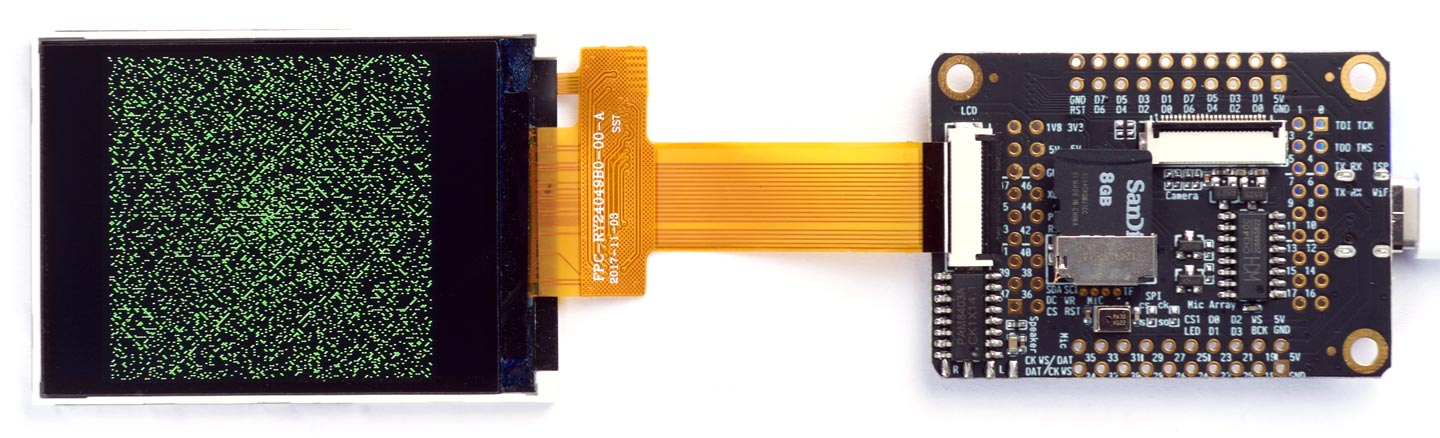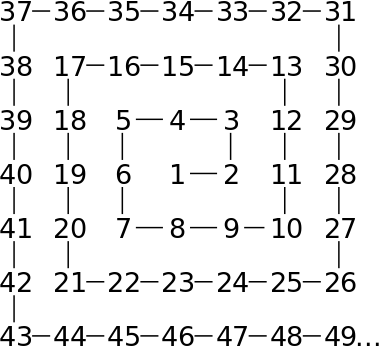## Prime number spiral

The following application demonstrates an application of uLisp's bit arrays to calculate and plot a prime number spiral, showing the pattern of prime numbers:This photo shows it running on a Sipeed MAiX One Dock, but you could also run it using the display on an Adafruit PyGamer or PyBadge.

It combines the technique of finding prime numbers known as the Sieve of Eratosthenes with a method of displaying the patterns in prime numbers called the Ulam Spiral.

This program was first published on the uLisp forum.

### Introduction

#### Sieve of Eratosthenes

The program uses a technique called the Sieve of Eratosthenes  to find all the prime numbers up to a certain number. The technique is attributed to the Greek mathematician Eratosthenes who lived in Cyrene between 276 and 194 BC.

The technique starts from a list of the integers from 2 up to some maximum number. 2 is the first number in the list, so this is the first prime number, and all multiples of 2 are crossed out.

The next highest number that hasn't been crossed out is 3; this is then the next prime number, and all multiples of 3 are crossed out.

The process is repeated until the end of the list is reached. The numbers left not crossed out in the list are then all the prime numbers up to the maximum number.

#### Ulam spiral

The Ulam spiral  is a graphical depiction of the prime numbers devised by mathematician Stanisław Ulam in 1963. It became popular after being featured in Martin Gardner's column Mathematical Games in Scientific American the following year.

It is constructed by first writing the positive integers in a square spiral:The prime numbers are then highlighted; in the program described here the prime numbers are shown as a green pixel against a black background.

When presented in a this way the pattern of primes looks far from random, and the primes appear to congregate along certain diagonal lines. These lines correspond to polynomials, and the Ulam Spiral has given rise to several unsolved problems in number theory.

### The program

The program demonstrates the use of bit arrays to implement the Sieve of Eratosthenes to find and display prime numbers in a Ulam spiral. The implementation of bit arrays on uLisp packs them as efficiently as possible, so they take up a factor of 32 less storage than the equivalent-sized integer array. Using a 57600-element bit array takes only 1815 Lisp objects, whereas if we were to use an integer array this would take 57618 objects, beyond what's available on many of the microcontrollers supported by uLisp.

Here's the program:

```(defun pspiral (width height &optional print)
(let* ((size (* height height))
(a (make-array size :element-type 'bit :initial-element 1))
(x0 (truncate width 2)) (y0 (truncate height 2))
(plotn (lambda (n colour)
(let ((c (spiral n)))
(draw-pixel
(+ (truncate width 2) (first c) -1)
(- (truncate height 2) (second c))
colour))))
(white #xFFFF)
(black 0)
(green #b0000011111100000)
(p 1) (n 0))
(fill-screen 0)
(fill-rect (truncate (- width height) 2) 0 height height)
(setf (aref a p) 0) (plotn p 0)
; Sieve of Eratosthenes
(loop
; Set p to next uncrossed number
(loop
(incf p)
(when (>= p size) (setq p nil) (return))
(when (= (aref a p) 1) (return)))
(when (null p) (return))
; Print next prime and plot it in green
(when print
(format t "~6d" p) (incf n)
(when (= n 20) (setq n 0) (format t "~%")))
(plotn p green)
; Cross out all increments of p
(let ((i p))
(loop
(incf i p)
(when (>= i size) (return))
(setf (aref a i) 0) (plotn i 0))))))```

The bit array is created with the statement:

`(make-array size :element-type 'bit :initial-element 1)`

The elements are initialised to the value 1, and are displayed in white. As numbers are found to be composite they are 'crossed out' by setting them to 0, and displayed in black.

The following function spiral converts a number n to a list of the x and y coordinates of its position on the spiral, with (0, 0) at the centre of the display :

```(defun spiral (n)
(let* ((k (ceiling (/ (1- (sqrt n)) 2)))
(j (1+ (* 2 k)))
(m (* j j))
(j (1- j)))
(cond
((>= n (- m j)) (list (- k (- m n)) (- k)))
((>= n (- (decf m j) j)) (list (- k) (+ (- k) (- m n))))
((>= n (- (decf m j) j)) (list (+ (- k) (- m n)) k))
(t (list k (- k (- m n j)))))))```

For example, the first number 1 is plotted at the centre of the display:

```> (spiral 1)
(0 0)```

and 10 is two positions to its right and one position down:

```> (spiral 10)
(2 -1)```

The local function plotn plots the pixel on the display corresponding to the number n:

```(plotn (lambda (n colour)
(let ((c (spiral n)))
(draw-pixel
(+ (truncate width 2) (first c) -1)
(- (truncate height 2) (second c))
colour))))```

To run the program give the command:

`(pspiral 360 240)`

You can also optionally print each prime to the serial monitor as it is found, with the command:

`(pspiral 360 240 t)`

If you've got a smaller display specify the dimensions as the arguments to pspiral; for example, on an Adafruit PyGamer or PyBadge use:

`(pspiral 160 120)`

Note that the program assumes the display is landscape format.

Here's the whole program in a single file: Prime number spiral.

1. ^ Sieve of Eratosthenes on Wikipedia.
2. ^ Ulam spiral on Wikipedia.
3. ^ Formula for spiral coordinates on Mathematics Stack Exchange.